## Introduction

Package MKmisc includes a collection of functions that I found useful in my daily work. It contains several functions for statistical data analysis; e.g. for sample size and power calculations, computation of confidence intervals, and generation of similarity matrices.

``````library(MKmisc)
``````

## Descriptive Statistics

### IQR

I implemented function IQrange before the standard function IQR gained the type argument. Since 2010 (r53643, r53644) the function is identical to function IQR.

``````x <- rnorm(100)
IQrange(x)
``````
``````##  1.317199
``````
``````IQR(x)
``````
``````##  1.317199
``````

It is also possible to compute a standardized version of the IQR leading to a normal-consistent estimate of the standard deviation.

``````sIQR(x)
``````
``````##  0.9764413
``````
``````sd(x)
``````
``````##  0.9627833
``````

### Five Number Summary

Tthere is a function that computes a so-called five number summary which in contrast to function fivenum uses the first and third quartile instead of the lower and upper hinge.

``````fiveNS(x)
``````
``````##     Minimum         25%      Median         75%     Maximum
## -2.58015060 -0.76205196 -0.04223123  0.55514728  1.95089785
``````

### Box- and Whisker-Plot

In contrast to the standard function boxplot which uses the lower and upper hinge for defining the box and the whiskers, the function qboxplot uses the first and third quartile.

``````x <- rt(10, df = 3)
par(mfrow = c(1,2))
qboxplot(x, main = "1st and 3rd quartile")
boxplot(x, main = "Lower and upper hinge")
``````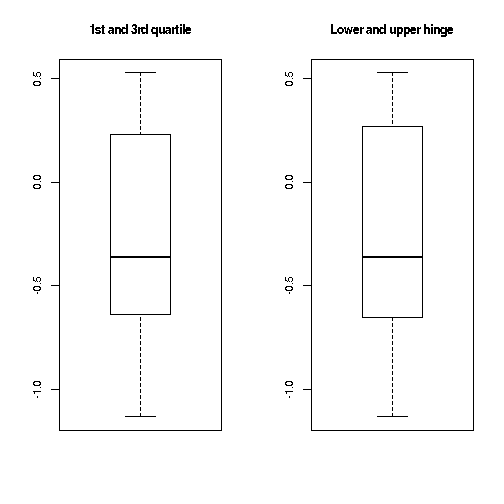The difference between the two versions often is hardly visible.

### OR, RR and Other Risk Measures

Given the incidence of the outcome of interest in the nonexposed (p0) and exposed (p1) group, several risk measures can be computed.

``````## Example from Wikipedia
risks(p0 = 0.4, p1 = 0.1)
``````
``````##        p0        p1        RR        OR       RRR       ARR       NNT
## 0.4000000 0.1000000 0.2500000 0.1666667 0.7500000 0.3000000 3.3333333
``````
``````risks(p0 = 0.4, p1 = 0.5)
``````
``````##    p0    p1    RR    OR   RRI   ARI   NNH
##  0.40  0.50  1.25  1.50  0.25  0.10 10.00
``````

Given p0 or p1 and OR, we can compute the respective RR.

``````or2rr(or = 1.5, p0 = 0.4)
``````
``````##  1.25
``````
``````or2rr(or = 1/6, p1 = 0.1)
``````
``````##  0.25
``````

### Generalized Logarithm

The generalized logarithm may be useful as a variance stabilizing transformation when also negative values are present.

``````curve(log, from = -3, to = 5)
``````
``````## Warning in log(x): NaNs wurden erzeugt
``````
``````curve(glog, from = -3, to = 5, add = TRUE, col = "orange")
legend("topleft", fill = c("black", "orange"), legend = c("log", "glog"))
``````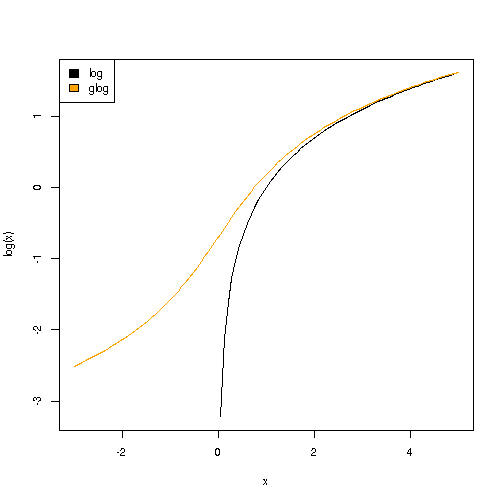As in case of function log there is also glog10 and glog2.

``````curve(log10(x), from = -3, to = 5)
``````
``````## Warning in eval(expr, envir = ll, enclos = parent.frame()): NaNs wurden
## erzeugt
``````
``````curve(glog10(x), from = -3, to = 5, add = TRUE, col = "orange")
legend("topleft", fill = c("black", "orange"), legend = c("log10", "glog10"))
``````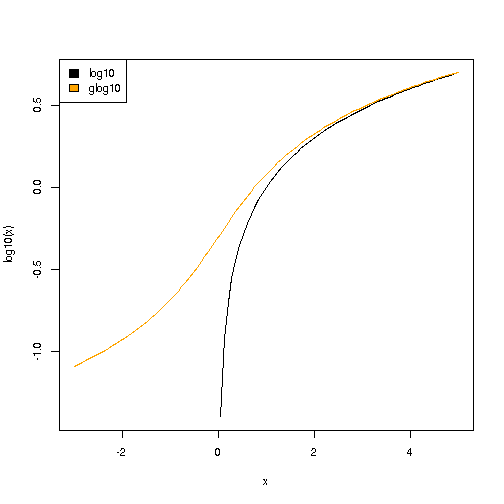There are also functions that compute the inverse of the generalized logarithm.

``````inv.glog(glog(10))
``````
``````##  10
``````
``````inv.glog(glog(10, base = 3), base = 3)
``````
``````##  10
``````
``````inv.glog10(glog10(10))
``````
``````##  10
``````
``````inv.glog2(glog2(10))
``````
``````##  10
``````

### Simulate Correlated Variables

To demonstrate Pearson correlation in my lectures, I have written this simple function to simulate correlated variables and to generate a scatter plot of the data.

``````res <- simCorVars(n = 500, r = 0.8)
``````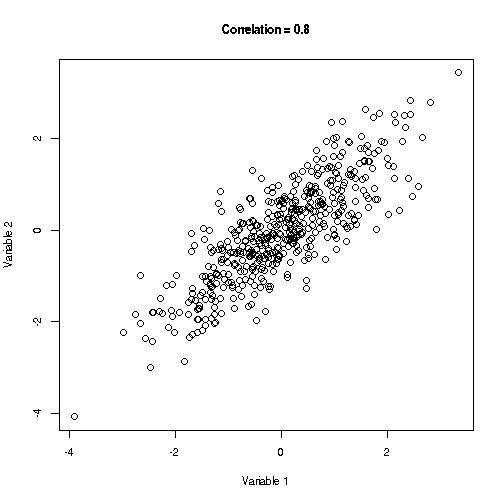``````cor(res\$Var1, res\$Var2)
``````
``````##  0.8210754
``````

### Plot TSH, fT3 and fT4 Values

The thyroid function is usually investigated by determining the values of TSH, fT3 and fT4. The function thyroid can be used to visualize the measured values as relative values with respect to the provided reference ranges.

``````thyroid(TSH = 1.5, fT3 = 2.5, fT4 = 14, TSHref = c(0.2, 3.0),
fT3ref = c(1.7, 4.2), fT4ref = c(7.6, 15.0))
``````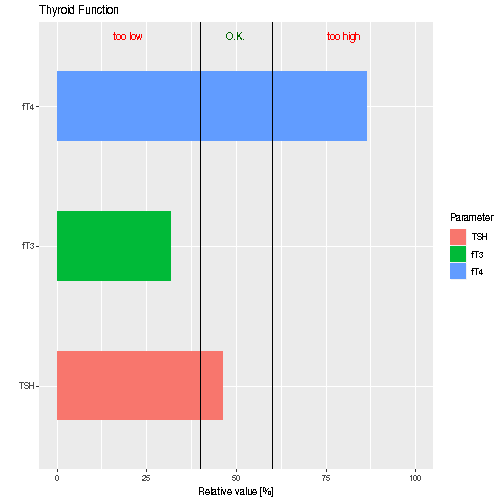### Generalized and Negative Logarithm as Transformations

We can use the generalized logarithm for transforming the axes in ggplot2 plots.

``````library(ggplot2)
data(mpg)
p1 <- ggplot(mpg, aes(displ, hwy)) + geom_point()
p1
``````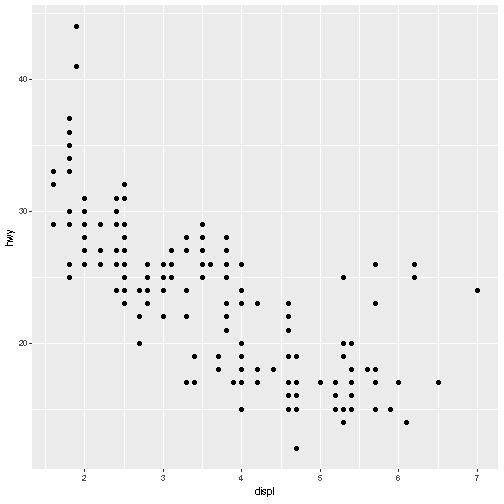``````p1 + scale_x_log10()
``````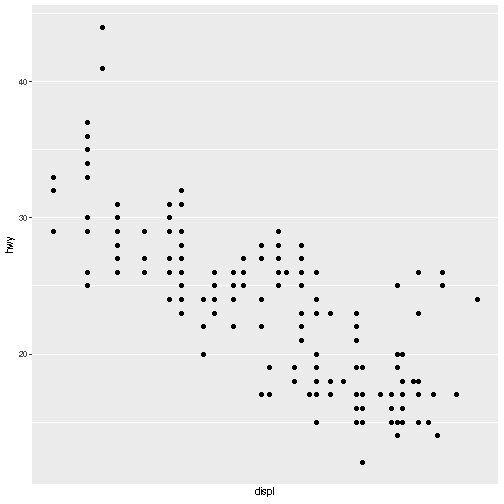``````p1 + scale_x_glog10()
``````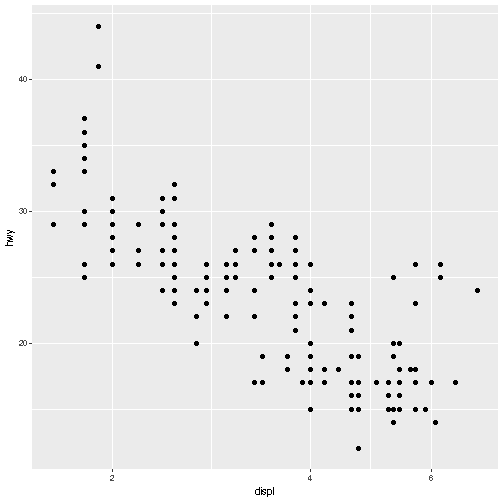``````p1 + scale_y_log10()
``````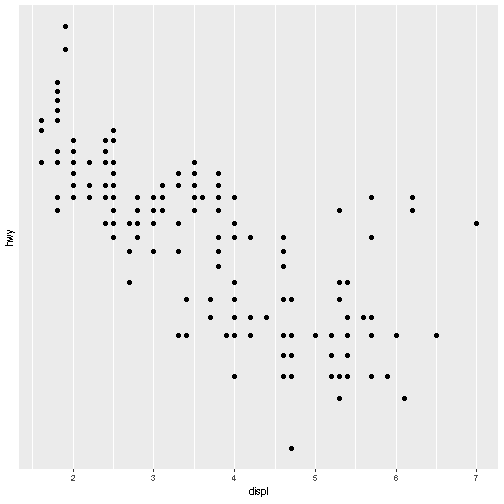``````p1 + scale_y_glog10()
``````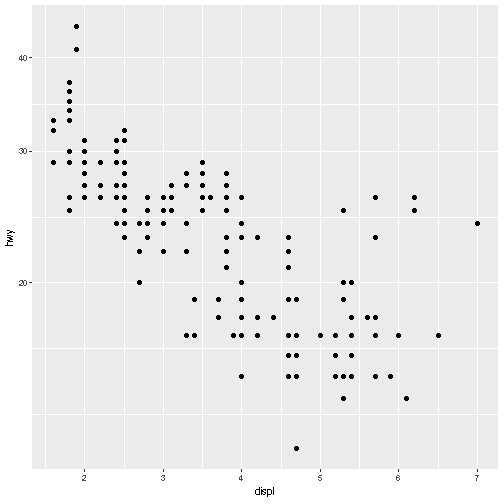The negative logrithm is for instance useful for displaying p values. The interesting values are on the top. This is for instance used in a so-called volcano plot.

``````x <- matrix(rnorm(1000, mean = 10), nrow = 10)
g1 <- rep("control", 10)
y1 <- matrix(rnorm(500, mean = 11.25), nrow = 10)
y2 <- matrix(rnorm(500, mean = 9.75), nrow = 10)
g2 <- rep("treatment", 10)
group <- factor(c(g1, g2))
Data <- rbind(x, cbind(y1, y2))
pvals <- apply(Data, 2, function(x, group) t.test(x ~ group)\$p.value,
group = group)
## compute log-fold change
logfc <- function(x, group){
res <- tapply(x, group, mean)
log2(res/res)
}
lfcs <- apply(Data, 2, logfc, group = group)
ps <- data.frame(pvals = pvals, logfc = lfcs)
ggplot(ps, aes(x = logfc, y = pvals)) + geom_point() +
geom_hline(yintercept = 0.05) + scale_y_neglog10() +
geom_vline(xintercept = c(-0.1, 0.1)) + xlab("log-fold change") +
ylab("-log10(p value)") + ggtitle("A Volcano Plot")
``````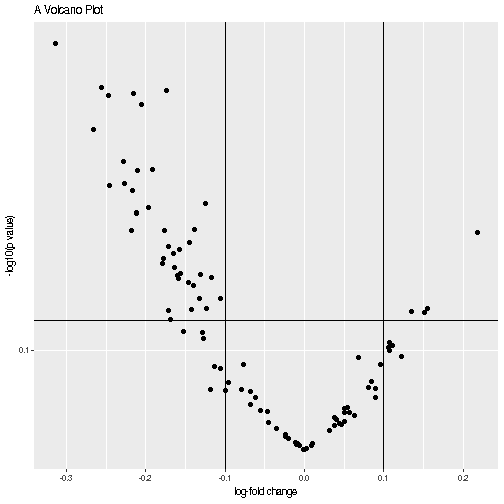### Change Data from Wide to Long

Often it's better to have the data in a long format than in a wide format; e.g., when plotting with package ggplot2. The necessary transformation can be done with function melt.long.

``````library(ggplot2)
## some random data
test <- data.frame(x = rnorm(10), y = rnorm(10), z = rnorm(10))
test.long <- melt.long(test)
test.long
``````
``````##           value variable
## x1  -0.62371359        x
## x2  -0.33997922        x
## x3   1.29334571        x
## x4  -0.07293021        x
## x5   0.17920020        x
## x6   0.31505210        x
## x7  -0.48242623        x
## x8  -1.65758466        x
## x9   1.62418684        x
## x10  0.08677947        x
## y1  -1.31329893        y
## y2   1.55187973        y
## y3  -1.02227966        y
## y4   2.04223111        y
## y5   0.35003686        y
## y6   1.09863719        y
## y7  -1.41457070        y
## y8   0.33041160        y
## y9   1.64109960        y
## y10 -0.61707783        y
## z1   0.29128488        z
## z2  -0.03122938        z
## z3  -0.46421121        z
## z4  -1.05047272        z
## z5  -0.90037359        z
## z6  -0.05956801        z
## z7  -0.62382519        z
## z8   0.83701799        z
## z9  -1.09824905        z
## z10 -1.96650936        z
``````
``````ggplot(test.long, aes(x = variable, y = value)) +
geom_boxplot(aes(fill = variable))
``````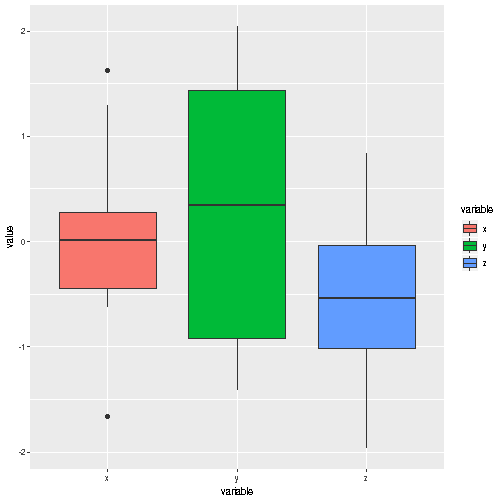``````## introducing an additional grouping variable
group <- factor(rep(c("a","b"), each = 5))
test.long.gr <- melt.long(test, select = 1:2, group = group)
test.long.gr
``````
``````##     group       value variable
## x1      a -0.62371359        x
## x2      a -0.33997922        x
## x3      a  1.29334571        x
## x4      a -0.07293021        x
## x5      a  0.17920020        x
## x6      b  0.31505210        x
## x7      b -0.48242623        x
## x8      b -1.65758466        x
## x9      b  1.62418684        x
## x10     b  0.08677947        x
## y1      a -1.31329893        y
## y2      a  1.55187973        y
## y3      a -1.02227966        y
## y4      a  2.04223111        y
## y5      a  0.35003686        y
## y6      b  1.09863719        y
## y7      b -1.41457070        y
## y8      b  0.33041160        y
## y9      b  1.64109960        y
## y10     b -0.61707783        y
``````
``````ggplot(test.long.gr, aes(x = variable, y = value, fill = group)) +
geom_boxplot()
``````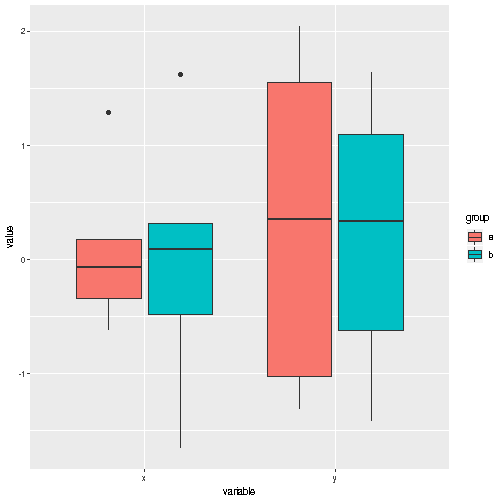## Confidence Intervals

### Binomial Proportion

There are several functions for computing confidence intervals. We can compute 10 different confidence intervals for binomial proportions; e.g.

``````## default: "wilson"
binomCI(x = 12, n = 50)
``````
``````##
##  wilson confidence interval
##
## 95 percent confidence interval:
##          2.5 %    97.5 %
## prob 0.1429739 0.3741268
##
## sample estimates:
## prob
## 0.24
``````
``````## Clopper-Pearson interval
binomCI(x = 12, n = 50, method = "clopper-pearson")
``````
``````##
##  clopper-pearson confidence interval
##
## 95 percent confidence interval:
##          2.5 %    97.5 %
## prob 0.1306099 0.3816907
##
## sample estimates:
## prob
## 0.24
``````
``````## identical to
binom.test(x = 12, n = 50)\$conf.int
``````
``````##  0.1306099 0.3816907
## attr(,"conf.level")
##  0.95
``````

For all intervals implemented see the help page of function binomCI.

### Mean and SD

We can compute confidence intervals for mean and SD of a normal distribution.

``````x <- rnorm(50, mean = 2, sd = 3)
## mean and SD unknown
normCI(x)
``````
``````##
##  Exact confidence interval(s)
##
## 95 percent confidence intervals:
##         2.5 %   97.5 %
## mean 1.225135 2.613010
## sd   2.039675 3.042744
##
## sample estimates:
##     mean       sd
## 1.919072 2.441748
``````
``````## SD known
normCI(x, sd = 3)
``````
``````##
##  Exact confidence interval(s)
##
## 95 percent confidence interval:
##        2.5 %   97.5 %
## mean 1.08753 2.750615
##
## sample estimates:
##     mean
## 1.919072
``````
``````## mean known
normCI(x, mean = 2)
``````
``````##
##  Exact confidence interval(s)
##
## 95 percent confidence interval:
##       2.5 %   97.5 %
## sd 2.039675 3.042744
##
## sample estimates:
##       sd
## 2.441748
``````

``````x <- rexp(100, rate = 0.5)
## exact
quantileCI(x = x, prob = 0.95)
``````
``````##
##  exact confidence interval
##
## 95.14464 percent confidence interval:
##                 lower upper
## 95 % quantile 4.76488  6.89
##
## sample estimates:
## 95 % quantile
##      5.313751
``````
``````## asymptotic
quantileCI(x = x, prob = 0.95, method = "asymptotic")
``````
``````##
##  asymptotic confidence interval
##
## 95 percent confidence interval:
##                 2.5 %   97.5 %
## 95 % quantile 4.76488 8.585352
##
## sample estimates:
## 95 % quantile
##      5.313751
``````

Next, we consider the median.

``````## exact
medianCI(x = x)
``````
``````##
##  exact confidence interval
##
## 95.23684 percent confidence intervals:
##            lower    upper
## median 0.9494647 1.822209
## median 1.1644517 1.978267
##
## sample estimates:
## median
## 1.4273
``````
``````## asymptotic
medianCI(x = x, method = "asymptotic")
``````
``````##
##  asymptotic confidence interval
##
## 95 percent confidence interval:
##           2.5 %   97.5 %
## median 1.091951 1.842177
##
## sample estimates:
## median
## 1.4273
``````

It often happens that quantile confidence intervals are not unique. Here the minimum length interval might be of interest.

``````medianCI(x = x, minLength = TRUE)
``````
``````##
##  minimum length exact confidence interval
##
## 95.23684 percent confidence interval:
##           lower    upper
## median 1.164452 1.978267
##
## sample estimates:
## median
## 1.4273
``````

Finally, we take a look at MAD (median absolute deviation) where by default the standardized MAD is used (see function mad).

``````## exact
``````
``````##
##  exact confidence interval
##
## 95.23684 percent confidence intervals:
##         lower    upper
##
## sample estimates:
## 1.365382
``````
``````## aysymptotic
madCI(x = x, method = "asymptotic")
``````
``````##
##  asymptotic confidence interval
##
## 95 percent confidence interval:
##        2.5 %   97.5 %
##
## sample estimates:
## 1.365382
``````
``````## unstandardized
madCI(x = x, constant = 1)
``````
``````##
##  exact confidence interval
##
## 95.23684 percent confidence intervals:
##         lower    upper
##
## sample estimates:
## 0.9209378
``````

### Relative Risk

There is also a function for computing an approximate confidence interval for the relative risk (RR).

``````## Example from Wikipedia
rrCI(a = 15, b = 135, c = 100, d = 150)
``````
``````##
##  asymptotic confidence interval
##
## 95 percent confidence interval:
##                   2.5 %    97.5 %
## relative risk 0.1510993 0.4136353
##
## sample estimates:
## relative risk
##          0.25
``````
``````rrCI(a = 75, b = 75, c = 100, d = 150)
``````
``````##
##  asymptotic confidence interval
##
## 95 percent confidence interval:
##                 2.5 %  97.5 %
## relative risk 1.00256 1.55851
##
## sample estimates:
## relative risk
##          1.25
``````

## AUC

### Estimation

There are two functions that can be used to calculate and test AUC values. First function AUC, which computes the area under the receiver operating characteristic curve (AUC under ROC curve) using the connection of AUC to the Wilcoxon rank sum test. We use some random data and groups to demonstrate the use of this function.

``````x <- c(runif(50, max = 0.6), runif(50, min = 0.4))
g <- c(rep(0, 50), rep(1, 50))
AUC(x, group = g)
``````
``````##  0.9412
``````

Sometimes the labels of the group should be switched to avoid an AUC smaller than 0.5, which represents a result worse than a pure random choice.

``````g <- c(rep(1, 50), rep(0, 50))
AUC(x, group = g)
``````
``````## Warning in AUC(x, group = g): The computed AUC value 0.0588 will be
## replaced by 0.9412 which can be achieved be interchanging the sample
## labels!
``````
``````##  0.9412
``````
``````## no switching
AUC(x, group = g, switchAUC = FALSE)
``````
``````##  0.0588
``````

### Testing

We can also perform statistical tests for AUC. First, the one-sample test which corresponds to the Wilcoxon signed rank test.

``````g <- c(rep(0, 50), rep(1, 50))
AUC.test(pred1 = x, lab1 = g)
``````
``````## \$Variable1
##        AUC         SE     low CI      up CI
## 0.94120000 0.02466384 0.89285976 0.98954024
##
## \$Test
##
##  Wilcoxon rank sum test with continuity correction
##
## data:  0 and 1
## W = 147, p-value = 2.951e-14
## alternative hypothesis: true AUC is not equal to 0.5
``````

We can also compare two AUC using the test of Hanley and McNeil (1982).

``````x2 <- c(runif(50, max = 0.7), runif(50, min = 0.3))
g2 <- c(rep(0, 50), rep(1, 50))
AUC.test(pred1 = x, lab1 = g, pred2 = x2, lab2 = g2)
``````
``````## \$Variable1
##        AUC         SE     low CI      up CI
## 0.94120000 0.02466384 0.89285976 0.98954024
##
## \$Variable2
##        AUC         SE     low CI      up CI
## 0.85520000 0.03841056 0.77991668 0.93048332
##
## \$Test
##
##  Hanley and McNeil test for two AUCs
##
## data:  x and x2
## z = 1.884, p-value = 0.05956
## alternative hypothesis: true difference in AUC is not equal to 0
## 95 percent confidence interval:
##  -0.003467074  0.175467074
## sample estimates:
## Difference in AUC
##             0.086
``````

### Pairwise

There is also a function for pairwise comparison if there are more than two groups.

``````x3 <- c(x, x2)
g3 <- c(g, c(rep(2, 50), rep(3, 50)))
pairwise.auc(x = x3, g = g3)
``````
``````## Warning in AUC(xi, xj): The computed AUC value 0.0588 will be replaced by
## 0.9412 which can be achieved be interchanging the sample labels!
``````
``````## Warning in AUC(xi, xj): The computed AUC value 0.4432 will be replaced by
## 0.5568 which can be achieved be interchanging the sample labels!
``````
``````## Warning in AUC(xi, xj): The computed AUC value 0.0956 will be replaced by
## 0.9044 which can be achieved be interchanging the sample labels!
``````
``````## Warning in AUC(xi, xj): The computed AUC value 0.1448 will be replaced by
## 0.8552 which can be achieved be interchanging the sample labels!
``````
``````## 0 vs 1 0 vs 2 0 vs 3 1 vs 2 1 vs 3 2 vs 3
## 0.9412 0.5568 0.9044 0.9036 0.6028 0.8552
``````

In addition to the pairwise.auc there are further functions for pairwise comparisons.

## Pairwise Comparisons

Often we are in a situation that we want to compare more than two groups pairwise.

### FC and logFC

In the analysis of omics data, the FC or logFC are important measures and are often used in combination with (adjusted) p values.

``````x <- rnorm(100) ## assumed as log-data
g <- factor(sample(1:4, 100, replace = TRUE))
levels(g) <- c("a", "b", "c", "d")
## modified FC
pairwise.fc(x, g)
``````
``````##    a vs b    a vs c    a vs d    b vs c    b vs d    c vs d
## -1.086272 -1.089956 -1.060579 -1.003391  1.024225  1.027699
``````
``````## "true" FC
pairwise.fc(x, g, mod.fc = FALSE)
``````
``````##    a vs b    a vs c    a vs d    b vs c    b vs d    c vs d
## 0.9205799 0.9174684 0.9428809 0.9966200 1.0242249 1.0276985
``````
``````## without any transformation
pairwise.logfc(x, g)
``````
``````##      a vs b      a vs c      a vs d      b vs c      b vs d      c vs d
## -0.11938509 -0.12426963 -0.08485254 -0.00488454  0.03453255  0.03941709
``````

The function returns a modified FC. That is, if the FC is smaller than 1 it is transformed to -1/FC. One can also use other functions than the mean for the aggregation of the data.

``````pairwise.logfc(x, g, ave = median)
``````
``````##      a vs b      a vs c      a vs d      b vs c      b vs d      c vs d
## -0.53454504 -0.06060438 -0.12336783  0.47394066  0.41117721 -0.06276345
``````

### Arbitrary Criteria

Furthermore, function pairwise.fun enables the application of arbitrary functions for pairwise comparisons.

``````pairwise.wilcox.test(airquality\$Ozone, airquality\$Month,
``````
``````## Warning in wilcox.test.default(xi, xj, paired = paired, ...): cannot
## compute exact p-value with ties

## Warning in wilcox.test.default(xi, xj, paired = paired, ...): cannot
## compute exact p-value with ties

## Warning in wilcox.test.default(xi, xj, paired = paired, ...): cannot
## compute exact p-value with ties

## Warning in wilcox.test.default(xi, xj, paired = paired, ...): cannot
## compute exact p-value with ties

## Warning in wilcox.test.default(xi, xj, paired = paired, ...): cannot
## compute exact p-value with ties

## Warning in wilcox.test.default(xi, xj, paired = paired, ...): cannot
## compute exact p-value with ties

## Warning in wilcox.test.default(xi, xj, paired = paired, ...): cannot
## compute exact p-value with ties

## Warning in wilcox.test.default(xi, xj, paired = paired, ...): cannot
## compute exact p-value with ties

## Warning in wilcox.test.default(xi, xj, paired = paired, ...): cannot
## compute exact p-value with ties

## Warning in wilcox.test.default(xi, xj, paired = paired, ...): cannot
## compute exact p-value with ties
``````
``````##
##  Pairwise comparisons using Wilcoxon rank sum test
##
## data:  airquality\$Ozone and airquality\$Month
##
##   5       6       7       8
## 6 0.19250 -       -       -
## 7 3e-05   0.01414 -       -
## 8 0.00012 0.02591 0.86195 -
## 9 0.11859 0.95887 0.00074 0.00325
##
## P value adjustment method: none
``````
``````## To avoid the warnings
library(exactRankTests)
``````
``````##  Package 'exactRankTests' is no longer under development.
``````
``````pairwise.fun(airquality\$Ozone, airquality\$Month,
fun = function(x, y) wilcox.exact(x, y)\$p.value)
``````
``````##       5 vs 6       5 vs 7       5 vs 8       5 vs 9       6 vs 7
## 1.897186e-01 1.087205e-05 6.108735e-05 1.171796e-01 1.183753e-02
##       6 vs 8       6 vs 9       7 vs 8       7 vs 9       8 vs 9
## 2.333564e-02 9.528659e-01 8.595683e-01 5.281796e-04 2.714694e-03
``````

## Binary Classification

### PPV and NPV

In case of medical diagnostic tests, usually sensitivity and specificity of the tests are known and there is also at least a rough estimate of the prevalence of the tested disease. In the practival application, the positive predictive value (PPV) and the negative predictive value are of crucial importance.

``````## Example: HIV test
## 1. ELISA screening test (4th generation)
predValues(sens = 0.999, spec = 0.998, prev = 0.001)
``````
``````##       PPV       NPV
## 0.3333333 0.9999990
``````
``````## 2. Western-Plot confirmation test
predValues(sens = 0.998, spec = 0.999996, prev = 1/3)
``````
``````##      PPV      NPV
## 0.999992 0.999001
``````

### Performance Measures

In the development of diagnostic tests and more general in binary classification a variety of performance measures can be found in literature. Function perfMeasures computes several of them.

``````## example from dataset infert
fit <- glm(case ~ spontaneous+induced, data = infert, family = binomial())
pred <- predict(fit, type = "response")

## with group numbers
perfMeasures(pred, truth = infert\$case, namePos = 1)
``````
``````##                                       Measure Value
## 1                              accuracy (ACC) 0.714
## 2  probabiliy of correct classification (PCC) 0.714
## 3     probability of missclassification (PMC) 0.286
## 4                                  error rate 0.286
## 5                                 sensitivity 0.337
## 6                                 specificity 0.903
## 7                                  prevalence 0.335
## 8                    balanced accuracy (BACC) 0.620
## 9                                informedness 0.240
## 10                       Youden's J statistic 0.240
## 11            positive predictive value (PPV) 0.636
## 12            negative predictive value (NPV) 0.730
## 13                                 markedness 0.367
## 14                                   F1 score 0.441
## 15    Matthews' correlation coefficient (MCC) 0.297
## 16         proportion of positive predictions 0.177
## 17                          expected accuracy 0.607
## 18                  Cohen's kappa coefficient 0.272
## 19             area under the ROC curve (AUC) 0.729
## 20                                 Gini index 0.457
## 21                                Brier score 0.191
## 22                       positive Brier score 0.361
## 23                       negative Brier score 0.106
## 24                       balanced Brier score 0.233
``````
``````## with group names
my.case <- factor(infert\$case, labels = c("control", "case"))
perfMeasures(pred, truth = my.case, namePos = "case")
``````
``````##                                       Measure Value
## 1                              accuracy (ACC) 0.714
## 2  probabiliy of correct classification (PCC) 0.714
## 3     probability of missclassification (PMC) 0.286
## 4                                  error rate 0.286
## 5                                 sensitivity 0.337
## 6                                 specificity 0.903
## 7                                  prevalence 0.335
## 8                    balanced accuracy (BACC) 0.620
## 9                                informedness 0.240
## 10                       Youden's J statistic 0.240
## 11            positive predictive value (PPV) 0.636
## 12            negative predictive value (NPV) 0.730
## 13                                 markedness 0.367
## 14                                   F1 score 0.441
## 15    Matthews' correlation coefficient (MCC) 0.297
## 16         proportion of positive predictions 0.177
## 17                          expected accuracy 0.607
## 18                  Cohen's kappa coefficient 0.272
## 19             area under the ROC curve (AUC) 0.729
## 20                                 Gini index 0.457
## 21                                Brier score 0.191
## 22                       positive Brier score 0.361
## 23                       negative Brier score 0.106
## 24                       balanced Brier score 0.233
``````

### Optimal Cutoff

The function optCutoff computes the optimal cutoff for various performance weasures for binary classification. More precisely, all performance measures that are implemented in function perfMeasures.

``````## example from dataset infert
fit <- glm(case ~ spontaneous+induced, data = infert, family = binomial())
pred <- predict(fit, type = "response")
optCutoff(pred, truth = infert\$case, namePos = 1)
``````
``````##      Optimal Cut-off Youden's J statistic
##            0.3034415            0.3470000
``````
``````optCutoff(pred, truth = infert\$case, namePos = 1,
perfMeasure = "balanced Brier score", max = FALSE)
``````
``````##      Optimal Cut-off balanced Brier score
##            0.1434415            0.2330000
``````
``````optCutoff(pred, truth = infert\$case, namePos = 1,
perfMeasure = "area under the ROC curve (AUC)")
``````
``````##                Optimal Cut-off area under the ROC curve (AUC)
##                      0.1434415                      0.7290000
``````

### Hosmer-Lemeshow and le Cessie-van Houwelingen-Copas-Hosmer

These tests are used to investigate the goodness of fit in logistic regression.

``````## Hosmer-Lemeshow goodness of fit tests for C and H statistic
HLgof.test(fit = pred, obs = infert\$case)
``````
``````## Warning in HLgof.test(fit = pred, obs = infert\$case): Found only 6
## different groups for Hosmer-Lemesho C statistic.
``````
``````## \$C
##
##  Hosmer-Lemeshow C statistic
##
## data:  pred and infert\$case
## X-squared = 4.4272, df = 4, p-value = 0.3513
##
##
## \$H
##
##  Hosmer-Lemeshow H statistic
##
## data:  pred and infert\$case
## X-squared = 6.3168, df = 8, p-value = 0.6118
``````
``````## e Cessie-van Houwelingen-Copas-Hosmer global goodness of fit test
HLgof.test(fit = pred, obs = infert\$case,
X = model.matrix(case ~ spontaneous+induced, data = infert))
``````
``````## Warning in HLgof.test(fit = pred, obs = infert\$case, X = model.matrix(case
## ~ : Found only 6 different groups for Hosmer-Lemesho C statistic.
``````
``````## \$C
##
##  Hosmer-Lemeshow C statistic
##
## data:  pred and infert\$case
## X-squared = 4.4272, df = 4, p-value = 0.3513
##
##
## \$H
##
##  Hosmer-Lemeshow H statistic
##
## data:  pred and infert\$case
## X-squared = 6.3168, df = 8, p-value = 0.6118
##
##
## \$gof
##
##  le Cessie-van Houwelingen-Copas-Hosmer global goodness of fit
##  test
##
## data:  pred and infert\$case
## z = 1.7533, p-value = 0.07954
``````

### Sample Size Calculation

Given an expected sensitivity and specificity we can compute sample size, power, delta or significance level of diagnostic test.

``````## see n2 on page 1202 of Chu and Cole (2007)
power.diagnostic.test(sens = 0.99, delta = 0.14, power = 0.95) # 40
``````
``````##
##      Diagnostic test exact power calculation
##
##            sens = 0.99
##               n = 40
##              n1 = 40
##           delta = 0.14
##       sig.level = 0.05
##           power = 0.95
##            prev = NULL
##
## NOTE: n is number of cases, n1 is number of controls
``````
``````power.diagnostic.test(sens = 0.99, delta = 0.13, power = 0.95) # 43
``````
``````##
##      Diagnostic test exact power calculation
##
##            sens = 0.99
##               n = 43
##              n1 = 43
##           delta = 0.13
##       sig.level = 0.05
##           power = 0.95
##            prev = NULL
##
## NOTE: n is number of cases, n1 is number of controls
``````
``````power.diagnostic.test(sens = 0.99, delta = 0.12, power = 0.95) # 47
``````
``````##
##      Diagnostic test exact power calculation
##
##            sens = 0.99
##               n = 47
##              n1 = 47
##           delta = 0.12
##       sig.level = 0.05
##           power = 0.95
##            prev = NULL
##
## NOTE: n is number of cases, n1 is number of controls
``````

The sample size planning for developing binary classifiers in case of high dimensional data, we can apply function ssize.pcc, which is based on the probability of correct classification (PCC).

``````## see Table 2 of Dobbin et al. (2008)
g <- 0.1
fc <- 1.6
ssize.pcc(gamma = g, stdFC = fc, nrFeatures = 22000)
``````
``````##
##      Sample Size Planning for Developing Classifiers Using High Dimensional Data
##
##           gamma = 0.1
##            prev = 0.5
##      nrFeatures = 22000
##              n1 = 21
##              n2 = 21
##
## NOTE: n1 is number of cases, n2 is number of controls
``````

## Sample Size: Two Negative Binomial Rates

When we consider two negative binomial rates, we can compute sample size or power applying function power.nb.test.

``````## examples from Table III in Zhu and Lakkis (2014)
power.nb.test(mu0 = 5.0, RR = 2.0, theta = 1/0.5, duration = 1, power = 0.8, approach = 1)
``````
``````##
##      Power calculation for comparing two negative binomial rates
##
##               n = 22.37386
##              n1 = 22.37386
##             mu0 = 5
##              RR = 2
##           theta = 2
##        duration = 1
##       sig.level = 0.05
##           power = 0.8
##     alternative = two.sided
##
## NOTE: n = sample size of control group, n1 = sample size of treatment group
``````
``````power.nb.test(mu0 = 5.0, RR = 2.0, theta = 1/0.5, duration = 1, power = 0.8, approach = 2)
``````
``````##
##      Power calculation for comparing two negative binomial rates
##
##               n = 21.23734
##              n1 = 21.23734
##             mu0 = 5
##              RR = 2
##           theta = 2
##        duration = 1
##       sig.level = 0.05
##           power = 0.8
##     alternative = two.sided
##
## NOTE: n = sample size of control group, n1 = sample size of treatment group
``````
``````power.nb.test(mu0 = 5.0, RR = 2.0, theta = 1/0.5, duration = 1, power = 0.8, approach = 3)
``````
``````##
##      Power calculation for comparing two negative binomial rates
##
##               n = 20.85564
##              n1 = 20.85564
##             mu0 = 5
##              RR = 2
##           theta = 2
##        duration = 1
##       sig.level = 0.05
##           power = 0.8
##     alternative = two.sided
##
## NOTE: n = sample size of control group, n1 = sample size of treatment group
``````

## Multiple Imputation t-Test

Function mi.t.test can be used to compute a multiple imputation t-test by applying the approch of Rubin (1987) in combination with the adjustment of Barnard and Rubin (1999).

``````## Generate some data
set.seed(123)
x <- rnorm(25, mean = 1)
x[sample(1:25, 5)] <- NA
y <- rnorm(20, mean = -1)
y[sample(1:20, 4)] <- NA
pair <- c(rnorm(25, mean = 1), rnorm(20, mean = -1))
g <- factor(c(rep("yes", 25), rep("no", 20)))
D <- data.frame(ID = 1:45, variable = c(x, y), pair = pair, group = g)

## Use Amelia to impute missing values
library(Amelia)
``````
``````## Loading required package: Rcpp
``````
``````## ##
## ## Amelia II: Multiple Imputation
## ## (Version 1.7.5, built: 2018-05-07)
## ## Copyright (C) 2005-2018 James Honaker, Gary King and Matthew Blackwell
## ##
``````
``````res <- amelia(D, m = 10, p2s = 0, idvars = "ID", noms = "group")

## Per protocol analysis (Welch two-sample t-test)
t.test(variable ~ group, data = D)
``````
``````##
##  Welch Two Sample t-test
##
## data:  variable by group
## t = -6.136, df = 30.075, p-value = 9.442e-07
## alternative hypothesis: true difference in means is not equal to 0
## 95 percent confidence interval:
##  -2.719747 -1.361505
## sample estimates:
##  mean in group no mean in group yes
##         -1.262978          0.777648
``````
``````## Intention to treat analysis (Multiple Imputation Welch two-sample t-test)
mi.t.test(res\$imputations, x = "variable", y = "group")
``````
``````##
##  Multiple Imputation Welch Two Sample t-test
##
## data:  Variable variable: group no vs group yes
## t = -6.2127, df = 27.254, p-value = 1.162e-06
## alternative hypothesis: true difference in means is not equal to 0
## 95 percent confidence interval:
##  -2.673999 -1.346690
## sample estimates:
##  mean (no)    SD (no) mean (yes)   SD (yes)
## -1.2578644  1.1335313  0.7524799  0.9539785
``````
``````## Per protocol analysis (Two-sample t-test)
t.test(variable ~ group, data = D, var.equal = TRUE)
``````
``````##
##  Two Sample t-test
##
## data:  variable by group
## t = -6.2305, df = 34, p-value = 4.331e-07
## alternative hypothesis: true difference in means is not equal to 0
## 95 percent confidence interval:
##  -2.706232 -1.375020
## sample estimates:
##  mean in group no mean in group yes
##         -1.262978          0.777648
``````
``````## Intention to treat analysis (Multiple Imputation two-sample t-test)
mi.t.test(res\$imputations, x = "variable", y = "group", var.equal = TRUE)
``````
``````##
##  Multiple Imputation Two Sample t-test
##
## data:  Variable variable: group no vs group yes
## t = -6.2923, df = 30.34, p-value = 5.881e-07
## alternative hypothesis: true difference in means is not equal to 0
## 95 percent confidence interval:
##  -2.662526 -1.358163
## sample estimates:
##  mean (no)    SD (no) mean (yes)   SD (yes)
## -1.2578644  1.1335313  0.7524799  0.9539785
``````
``````## Specifying alternatives
mi.t.test(res\$imputations, x = "variable", y = "group", alternative = "less")
``````
``````##
##  Multiple Imputation Welch Two Sample t-test
##
## data:  Variable variable: group no vs group yes
## t = -6.2127, df = 27.254, p-value = 5.811e-07
## alternative hypothesis: true difference in means is less than 0
## 95 percent confidence interval:
##       -Inf -1.459366
## sample estimates:
##  mean (no)    SD (no) mean (yes)   SD (yes)
## -1.2578644  1.1335313  0.7524799  0.9539785
``````
``````mi.t.test(res\$imputations, x = "variable", y = "group", alternative = "greater")
``````
``````##
##  Multiple Imputation Welch Two Sample t-test
##
## data:  Variable variable: group no vs group yes
## t = -6.2127, df = 27.254, p-value = 1
## alternative hypothesis: true difference in means is greater than 0
## 95 percent confidence interval:
##  -2.561323       Inf
## sample estimates:
##  mean (no)    SD (no) mean (yes)   SD (yes)
## -1.2578644  1.1335313  0.7524799  0.9539785
``````
``````## One sample test
t.test(D\$variable[D\$group == "yes"])
``````
``````##
##  One Sample t-test
##
## data:  D\$variable[D\$group == "yes"]
## t = 3.796, df = 19, p-value = 0.001221
## alternative hypothesis: true mean is not equal to 0
## 95 percent confidence interval:
##  0.348868 1.206428
## sample estimates:
## mean of x
##  0.777648
``````
``````mi.t.test(res\$imputations, x = "variable", subset = D\$group == "yes")
``````
``````##
##  Multiple Imputation One Sample t-test
##
## data:  Variable variable
## t = 3.9439, df = 19.617, p-value = 0.0008272
## alternative hypothesis: true mean is not equal to 0
## 95 percent confidence interval:
##  0.3539882 1.1509716
## sample estimates:
##      mean        SD
## 0.7524799 0.9539785
``````
``````mi.t.test(res\$imputations, x = "variable", mu = -1, subset = D\$group == "yes",
alternative = "less")
``````
``````##
##  Multiple Imputation One Sample t-test
##
## data:  Variable variable
## t = 9.1851, df = 19.617, p-value = 1
## alternative hypothesis: true mean is less than -1
## 95 percent confidence interval:
##      -Inf 1.081861
## sample estimates:
##      mean        SD
## 0.7524799 0.9539785
``````
``````mi.t.test(res\$imputations, x = "variable", mu = -1, subset = D\$group == "yes",
alternative = "greater")
``````
``````##
##  Multiple Imputation One Sample t-test
##
## data:  Variable variable
## t = 9.1851, df = 19.617, p-value = 7.679e-09
## alternative hypothesis: true mean is greater than -1
## 95 percent confidence interval:
##  0.4230991       Inf
## sample estimates:
##      mean        SD
## 0.7524799 0.9539785
``````
``````## paired test
t.test(D\$variable, D\$pair, paired = TRUE)
``````
``````##
##  Paired t-test
##
## data:  D\$variable and D\$pair
## t = -0.84172, df = 35, p-value = 0.4057
## alternative hypothesis: true difference in means is not equal to 0
## 95 percent confidence interval:
##  -0.6224989  0.2575965
## sample estimates:
## mean of the differences
##              -0.1824512
``````
``````mi.t.test(res\$imputations, x = "variable", y = "pair", paired = TRUE)
``````
``````##
##  Multiple Imputation Paired t-test
##
## data:  Variables  variable and pair
## t = -1.1136, df = 39.142, p-value = 0.2723
## alternative hypothesis: true difference in means is not equal to 0
## 95 percent confidence interval:
##  -0.6063388  0.1757321
## sample estimates:
## mean of difference   SD of difference
##         -0.2153033          1.2970098
``````

## Omics Data

### Aggregating Technical Replicates

In case of omics experiments it is often the case that technical replicates are determined and hence it is part of the preprocessing of the raw data to aggregate these technical replicates. This is the purpose of function repMeans.

``````M <- matrix(rnorm(100), ncol = 5)
FL <- matrix(rpois(100, lambda = 10), ncol = 5) # only for this example
repMeans(x = M, flags = FL, use.flags = "max", ndups = 5, spacing = 4)
``````
``````## \$exprs
##            [,1]        [,2]       [,3]       [,4]        [,5]
## [1,]  0.5931505  0.28807059  1.1242150  0.2008174  0.16405926
## [2,] -0.3124689  0.97892357  1.0187999 -0.3327906  0.25880177
## [3,]  1.0452833 -0.07026051  0.4395632  0.7598702  0.08980247
## [4,]  1.8646156  2.41134608 -0.4825960  0.5007072 -0.15497574
##
## \$flags
##      [,1] [,2] [,3] [,4] [,5]
## [1,]   12   12   14   11   15
## [2,]   17   18   18   13   13
## [3,]   11   13   18   12   15
## [4,]   13   11   17   15   11
``````

### 1- and 2-Way ANOVA

Functions oneWayAnova and twoWayAnova return a function that can be used to perform a 1- or 2-way ANOVA, respectively.

``````af <- oneWayAnova(c(rep(1,5),rep(2,5)))
## p value
af(rnorm(10))
``````
``````##  0.3476601
``````
``````x <- matrix(rnorm(12*10), nrow = 10)
## 2-way ANOVA with interaction
af1 <- twoWayAnova(c(rep(1,6),rep(2,6)), rep(c(rep(1,3), rep(2,3)), 2))
## p values
apply(x, 1, af1)
``````
``````##                  [,1]       [,2]      [,3]      [,4]      [,5]      [,6]
## cov1        0.1711293 0.07492485 0.9386028 0.6927840 0.2599459 0.1376865
## cov2        0.9016391 0.25160300 0.4913217 0.3473045 0.6729475 0.5862574
## interaction 0.3794393 0.26662669 0.8074828 0.3609952 0.4734751 0.6822754
##                  [,7]      [,8]      [,9]     [,10]
## cov1        0.9746284 0.8330694 0.8136081 0.6695168
## cov2        0.1719117 0.5184855 0.7931330 0.6465271
## interaction 0.6330320 0.7424491 0.4388955 0.9517314
``````
``````## 2-way ANOVA without interaction
af2 <- twoWayAnova(c(rep(1,6),rep(2,6)), rep(c(rep(1,3), rep(2,3)), 2),
interaction = FALSE)
## p values
apply(x, 1, af2)
``````
``````##           [,1]       [,2]      [,3]      [,4]      [,5]      [,6]
## cov1 0.1641183 0.07659801 0.9349189 0.6906204 0.2453634 0.1176840
## cov2 0.9005539 0.25800967 0.4655965 0.3425853 0.6640486 0.5666643
##           [,7]      [,8]      [,9]     [,10]
## cov1 0.9734032 0.8237512 0.8094216 0.6497189
## cov2 0.1514584 0.4949762 0.7885011 0.6255718
``````

### Correlation Distance Matrix

In the analysis of omics data correlation and absolute correlation distance matrices are often used during quality control. Function corDist can compute the Pearson, Spearman, Kendall or Cosine sample correlation and absolute correlation as well as the minimum covariance determinant or the orthogonalized Gnanadesikan-Kettenring correlation and absolute correlation.

``````M <- matrix(rcauchy(1000), nrow = 5)
## Pearson
corDist(M)
``````
``````##           1         2         3         4
## 2 0.4218522
## 3 0.9975857 1.0128921
## 4 0.9955133 0.9952809 0.9935421
## 5 1.0111642 1.0432974 1.0062979 1.0300565
``````
``````## Spearman
corDist(M, method = "spearman")
``````
``````##           1         2         3         4
## 2 1.0664277
## 3 0.9433571 1.0764764
## 4 1.0368919 0.9780965 1.0462672
## 5 1.0992035 1.0681077 1.0188030 1.0048976
``````
``````## Minimum Covariance Determinant
corDist(M, method = "mcd")
``````
``````##           1         2         3         4
## 2 1.1085210
## 3 0.9553460 1.0797273
## 4 1.1421452 0.7740163 1.0252023
## 5 1.1121205 1.0678142 1.0464306 0.9864231
``````

In case of outliers the MAD may be useful as dispersion measure.

``````madMatrix(t(M))
``````
``````##          [,1]     [,2]     [,3]     [,4]     [,5]
## [1,] 0.000000 2.691921 2.934757 2.834333 2.832269
## [2,] 2.691921 0.000000 3.162959 2.468369 2.552503
## [3,] 2.934757 3.162959 0.000000 3.477265 3.314816
## [4,] 2.834333 2.468369 3.477265 0.000000 3.139659
## [5,] 2.832269 2.552503 3.314816 3.139659 0.000000
``````

### Similarity Matrices

First, we can plot a similarity matrix based on correlation.

``````M <- matrix(rnorm(1000), ncol = 20)
colnames(M) <- paste("Sample", 1:20)
M.cor <- cor(M)
corPlot(M.cor, minCor = min(M.cor), labels = colnames(M))
``````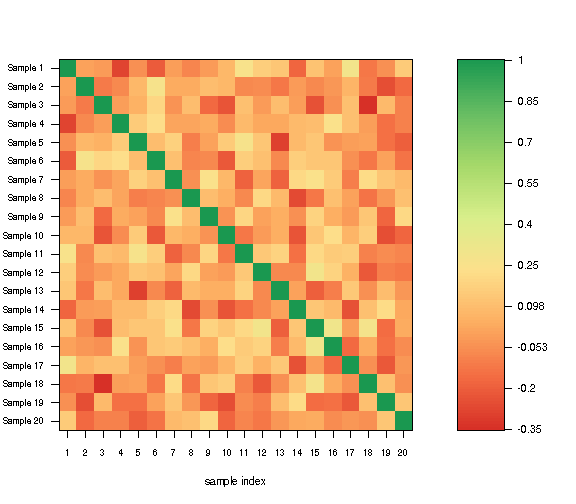Next, we can use the MAD.

``````## random data
x <- matrix(rnorm(1000), ncol = 10)
## outliers
x[1:20,5] <- x[1:20,5] + 10
``````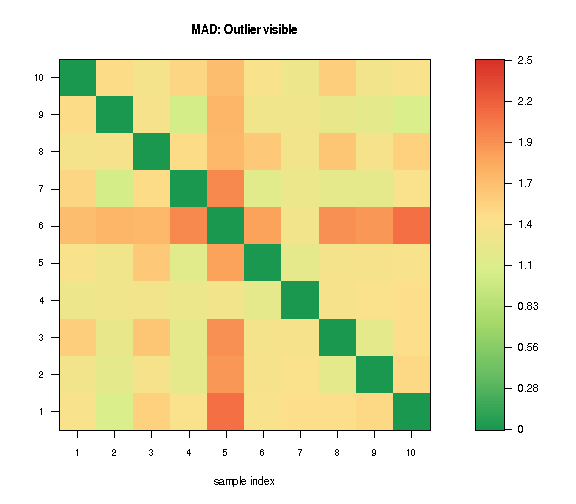``````## in contrast
corPlot(x, new = TRUE, minCor = -0.5, labels = TRUE,
``````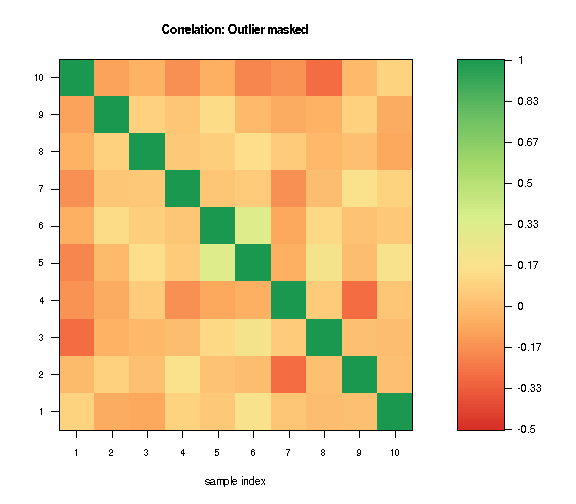### Colors for Heatmaps

Nowadays there are better solutions e.g. provided by Bioconductor package complexHeatmaps. This was my solution to get a better coloring of heatmaps.

``````## generate some random data
data.plot <- matrix(rnorm(100*50, sd = 1), ncol = 50)
colnames(data.plot) <- paste("patient", 1:50)
rownames(data.plot) <- paste("gene", 1:100)
data.plot[1:70, 1:30] <- data.plot[1:70, 1:30] + 3
data.plot[71:100, 31:50] <- data.plot[71:100, 31:50] - 1.4
data.plot[1:70, 31:50] <- rnorm(1400, sd = 1.2)
data.plot[71:100, 1:30] <- rnorm(900, sd = 1.2)
nrcol <- 128
library(gplots)
``````
``````##
## Attaching package: 'gplots'
``````
``````## The following object is masked from 'package:stats':
##
##     lowess
``````
``````library(RColorBrewer)
myCol <- rev(colorRampPalette(brewer.pal(10, "RdBu"))(nrcol))
heatmap.2(data.plot, col =  myCol, trace = "none", tracecol = "black",
main = "standard colors")
``````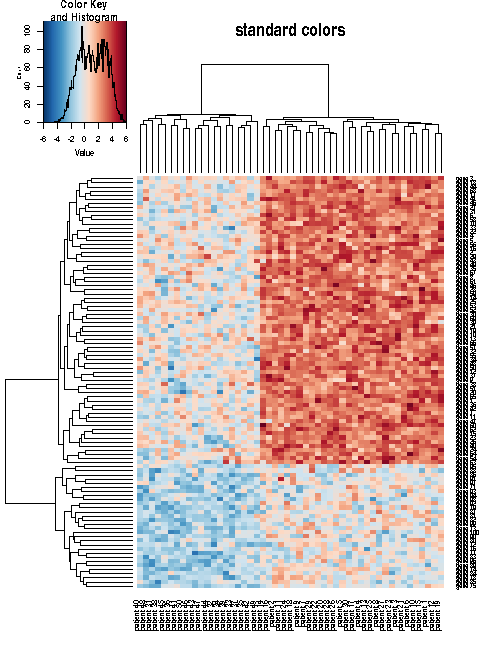``````farbe <- heatmapCol(data = data.plot, col = myCol,
lim = min(abs(range(data.plot)))-1)
heatmap.2(data.plot, col = farbe, trace = "none", tracecol = "black",
main = "heatmapCol colors")
``````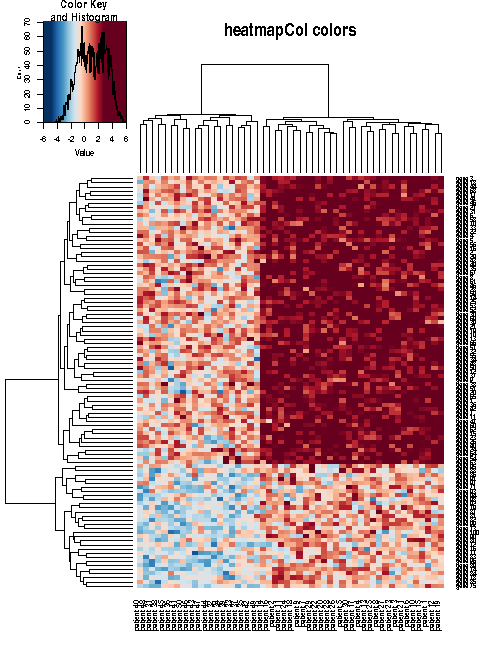## String Alignment

### String Distances

In Bioinformatics the (pairwise and multiple) alignment of strings is an important topic. For this one can use the distance or similarity between strings. The Hamming and the the Levenshtein (edit) distance are implemented in function stringDist.

``````x <- "GACGGATTATG"
y <- "GATCGGAATAG"
## Hamming distance
stringDist(x, y)
``````
``````##             GACGGATTATG
## GATCGGAATAG           3
``````
``````## Levenshtein distance
d <- stringDist(x, y)
d
``````
``````##             GACGGATTATG
## GATCGGAATAG           3
``````

In case of the Levenshtein (edit) distance, the respective scoring and traceback matrices are attached as attributes to the result.

``````attr(d, "ScoringMatrix")
``````
``````##     gap  G A T C G G A A T  A  G
## gap   0  1 2 3 4 5 6 7 8 9 10 11
## G     1  0 1 2 3 4 5 6 7 8  9 10
## A     2  1 0 1 2 3 4 5 6 7  8  9
## C     3  2 1 1 1 2 3 4 5 6  7  8
## G     4  3 2 2 2 1 2 3 4 5  6  7
## G     5  4 3 3 3 2 1 2 3 4  5  6
## A     6  5 4 4 4 3 2 1 2 3  4  5
## T     7  6 5 4 5 4 3 2 2 2  3  4
## T     8  7 6 5 5 5 4 3 3 2  3  4
## A     9  8 7 6 6 6 5 4 3 3  2  3
## T    10  9 8 7 7 7 6 5 4 3  3  3
## G    11 10 9 8 8 7 7 6 5 4  4  3
``````
``````attr(d, "TraceBackMatrix")
``````
``````##     gap     G     A     T      C        G      G     A     A      T
## gap "start" "i"   "i"   "i"    "i"      "i"    "i"   "i"   "i"    "i"
## G   "d"     "m"   "i"   "i"    "i"      "m/i"  "m/i" "i"   "i"    "i"
## A   "d"     "d"   "m"   "i"    "i"      "i"    "i"   "m/i" "m/i"  "i"
## C   "d"     "d"   "d"   "mm"   "m"      "i"    "i"   "i"   "i"    "i"
## G   "d"     "d/m" "d"   "d/mm" "d/mm"   "m"    "m/i" "i"   "i"    "i"
## G   "d"     "d/m" "d"   "d/mm" "d/mm"   "d/m"  "m"   "i"   "i"    "i"
## A   "d"     "d"   "d/m" "d/mm" "d/mm"   "d"    "d"   "m"   "m/i"  "i"
## T   "d"     "d"   "d"   "m"    "d/mm/i" "d"    "d"   "d"   "mm"   "m"
## T   "d"     "d"   "d"   "d/m"  "mm"     "d"    "d"   "d"   "d/mm" "m"
## A   "d"     "d"   "d/m" "d"    "d/mm"   "d/mm" "d"   "d/m" "m"    "d"
## T   "d"     "d"   "d"   "d/m"  "d/mm"   "d/mm" "d"   "d"   "d"    "m"
## G   "d"     "d/m" "d"   "d"    "d/mm"   "m"    "d/m" "d"   "d"    "d"
##     A      G
## gap "i"    "i"
## G   "i"    "m/i"
## A   "m/i"  "i"
## C   "i"    "i"
## G   "i"    "m/i"
## G   "i"    "m/i"
## A   "m/i"  "i"
## T   "i"    "i"
## T   "mm/i" "mm/i"
## A   "m"    "i"
## T   "d"    "mm"
## G   "d/mm" "m"
``````

The characters in the trace-back matrix reflect insertion of a gap in string y (d: deletion), match (m), mismatch (mm), and insertion of a gap in string x (i).

### String Similarities

The function stringSim computes the optimal alignment scores for global (Needleman-Wunsch) and local (Smith-Waterman) alignments with constant gap penalties. Scoring and trace-back matrix are again attached as attributes to the results.

``````## optimal global alignment score
d <- stringSim(x, y)
d
``````
``````##             GACGGATTATG
## GATCGGAATAG           6
``````
``````attr(d, "ScoringMatrix")
``````
``````##     gap  G  A  T  C  G  G  A  A  T   A   G
## gap   0 -1 -2 -3 -4 -5 -6 -7 -8 -9 -10 -11
## G    -1  1  0 -1 -2 -3 -4 -5 -6 -7  -8  -9
## A    -2  0  2  1  0 -1 -2 -3 -4 -5  -6  -7
## C    -3 -1  1  1  2  1  0 -1 -2 -3  -4  -5
## G    -4 -2  0  0  1  3  2  1  0 -1  -2  -3
## G    -5 -3 -1 -1  0  2  4  3  2  1   0  -1
## A    -6 -4 -2 -2 -1  1  3  5  4  3   2   1
## T    -7 -5 -3 -1 -2  0  2  4  4  5   4   3
## T    -8 -6 -4 -2 -2 -1  1  3  3  5   4   3
## A    -9 -7 -5 -3 -3 -2  0  2  4  4   6   5
## T   -10 -8 -6 -4 -4 -3 -1  1  3  5   5   5
## G   -11 -9 -7 -5 -5 -3 -2  0  2  4   4   6
``````
``````attr(d, "TraceBackMatrix")
``````
``````##     gap     G     A     T      C      G     G     A     A      T   A
## gap "start" "i"   "i"   "i"    "i"    "i"   "i"   "i"   "i"    "i" "i"
## G   "d"     "m"   "i"   "i"    "i"    "m/i" "m/i" "i"   "i"    "i" "i"
## A   "d"     "d"   "m"   "i"    "i"    "i"   "i"   "m/i" "m/i"  "i" "m/i"
## C   "d"     "d"   "d"   "mm"   "m"    "i"   "i"   "i"   "i"    "i" "i"
## G   "d"     "d/m" "d"   "d/mm" "d"    "m"   "m/i" "i"   "i"    "i" "i"
## G   "d"     "d/m" "d"   "d/mm" "d"    "d/m" "m"   "i"   "i"    "i" "i"
## A   "d"     "d"   "d/m" "d/mm" "d"    "d"   "d"   "m"   "m/i"  "i" "m/i"
## T   "d"     "d"   "d"   "m"    "d/i"  "d"   "d"   "d"   "mm"   "m" "i"
## T   "d"     "d"   "d"   "d/m"  "mm"   "d"   "d"   "d"   "d/mm" "m" "mm/i"
## A   "d"     "d"   "d/m" "d"    "d/mm" "d"   "d"   "d/m" "m"    "d" "m"
## T   "d"     "d"   "d"   "d/m"  "d/mm" "d"   "d"   "d"   "d"    "m" "d"
## G   "d"     "d/m" "d"   "d"    "d/mm" "m"   "d/m" "d"   "d"    "d" "d/mm"
##     G
## gap "i"
## G   "m/i"
## A   "i"
## C   "i"
## G   "m/i"
## G   "m/i"
## A   "i"
## T   "i"
## T   "mm/i"
## A   "i"
## T   "mm"
## G   "m"
``````
``````## optimal local alignment score
d <- stringSim(x, y, global = FALSE)
d
``````
``````##             GACGGATTATG
## GATCGGAATAG           6
``````
``````attr(d, "ScoringMatrix")
``````
``````##     gap G A T C G G A A T A G
## gap   0 0 0 0 0 0 0 0 0 0 0 0
## G     0 1 0 0 0 1 1 0 0 0 0 1
## A     0 0 2 1 0 0 0 2 1 0 1 0
## C     0 0 1 1 2 1 0 1 1 0 0 0
## G     0 1 0 0 1 3 2 1 0 0 0 1
## G     0 1 0 0 0 2 4 3 2 1 0 1
## A     0 0 2 1 0 1 3 5 4 3 2 1
## T     0 0 1 3 2 1 2 4 4 5 4 3
## T     0 0 0 2 2 1 1 3 3 5 4 3
## A     0 0 1 1 1 1 0 2 4 4 6 5
## T     0 0 0 2 1 0 0 1 3 5 5 5
## G     0 1 0 1 1 2 1 0 2 4 4 6
``````
``````attr(d, "TraceBackMatrix")
``````
``````##     gap     G        A           T           C        G
## gap "start" "i"      "i"         "i"         "i"      "i"
## G   "d"     "m"      "i/stop"    "stop"      "stop"   "m"
## A   "d"     "d/stop" "m"         "i"         "i/stop" "d/stop"
## C   "d"     "stop"   "d"         "mm"        "m"      "i"
## G   "d"     "m"      "d/i/stop"  "d/mm/stop" "d"      "m"
## G   "d"     "m"      "mm/i/stop" "stop"      "d/stop" "d/m"
## A   "d"     "d/stop" "m"         "i"         "i/stop" "d"
## T   "d"     "stop"   "d"         "m"         "i"      "i"
## T   "d"     "stop"   "d/stop"    "d/m"       "mm"     "mm/i"
## A   "d"     "stop"   "m"         "d"         "d/mm"   "mm"
## T   "d"     "stop"   "d/stop"    "m"         "i"      "d/mm/i/stop"
## G   "d"     "m"      "i/stop"    "d"         "mm"     "m"
##     G             A          A             T           A        G
## gap "i"           "i"        "i"           "i"         "i"      "i"
## G   "m"           "i/stop"   "stop"        "stop"      "stop"   "m"
## A   "d/mm/stop"   "m"        "m/i"         "i/stop"    "m"      "d/i/stop"
## C   "i/stop"      "d"        "mm"          "mm/i/stop" "d/stop" "mm/stop"
## G   "m/i"         "i"        "d/mm/i/stop" "mm/stop"   "stop"   "m"
## G   "m"           "i"        "i"           "i"         "i/stop" "m"
## A   "d"           "m"        "m/i"         "i"         "m/i"    "i"
## T   "d"           "d"        "mm"          "m"         "i"      "i"
## T   "d"           "d"        "d/mm"        "m"         "mm/i"   "mm/i"
## A   "d/mm/i/stop" "d/m"      "m"           "d"         "m"      "i"
## T   "mm/stop"     "d"        "d"           "m"         "d"      "mm"
## G   "m/i"         "d/i/stop" "d"           "d"         "d/mm"   "m"
``````

The entry stop indicates that the minimum similarity score has been reached.

### Optimal Alignment

Finally, the function traceBack computes an optimal global or local alignment based on a trace back matrix as provided by function stringDist or stringSim.

``````x <- "GACGGATTATG"
y <- "GATCGGAATAG"
## Levenshtein distance
d <- stringDist(x, y)
## optimal global alignment
traceBack(d)
``````
``````##    pairwise global alignment
## x' "GA-CGGATTATG"
## y' "GATCGGAATA-G"
``````
``````## Optimal global alignment score
d <- stringSim(x, y)
## optimal global alignment
traceBack(d)
``````
``````##    pairwise global alignment
## x' "GA-CGGATTATG"
## y' "GATCGGAATA-G"
``````
``````## Optimal local alignment score
d <- stringSim(x, y, global = FALSE)
## optimal local alignment
traceBack(d, global = FALSE)
``````
``````##    pairwise local alignment
## x' "GA-CGGATTA"
## y' "GATCGGAATA"
``````

## sessionInfo

``````sessionInfo()
``````
``````## R version 3.5.1 Patched (2018-07-24 r75008)
## Platform: x86_64-pc-linux-gnu (64-bit)
## Running under: Ubuntu 16.04.5 LTS
##
## Matrix products: default
## BLAS: /usr/lib/atlas-base/atlas/libblas.so.3.0
## LAPACK: /home/kohlm/RTOP/Rbranch/lib/libRlapack.so
##
## locale:
##   LC_CTYPE=de_DE.UTF-8       LC_NUMERIC=C
##   LC_TIME=de_DE.UTF-8        LC_COLLATE=C
##   LC_MONETARY=de_DE.UTF-8    LC_MESSAGES=de_DE.UTF-8
##   LC_PAPER=de_DE.UTF-8       LC_NAME=C
##  LC_MEASUREMENT=de_DE.UTF-8 LC_IDENTIFICATION=C
##
## attached base packages:
##  stats     graphics  grDevices utils     datasets  methods   base
##
## other attached packages:
##  RColorBrewer_1.1-2    gplots_3.0.1          Amelia_1.7.5
##  Rcpp_0.12.18          exactRankTests_0.8-29 ggplot2_3.0.0
##  MKmisc_1.1
##
## loaded via a namespace (and not attached):
##   pillar_1.3.0       compiler_3.5.1     DEoptimR_1.0-8
##   plyr_1.8.4         highr_0.7          bindr_0.1.1
##   bitops_1.0-6       tools_3.5.1        digest_0.6.15
##  evaluate_0.11      tibble_1.4.2       gtable_0.2.0
##  pkgconfig_2.0.1    rlang_0.2.1        bindrcpp_0.2.2
##  withr_2.1.2        dplyr_0.7.6        stringr_1.3.1
##  knitr_1.20         caTools_1.17.1.1   gtools_3.8.1
##  grid_3.5.1         tidyselect_0.2.4   glue_1.3.0
##  robustbase_0.93-2  R6_2.2.2           foreign_0.8-71
##  gdata_2.18.0       purrr_0.2.5        magrittr_1.5
##  scales_0.5.0       assertthat_0.2.0   colorspace_1.3-2
##  labeling_0.3       KernSmooth_2.23-15 stringi_1.2.4
##  lazyeval_0.2.1     munsell_0.5.0      crayon_1.3.4
``````